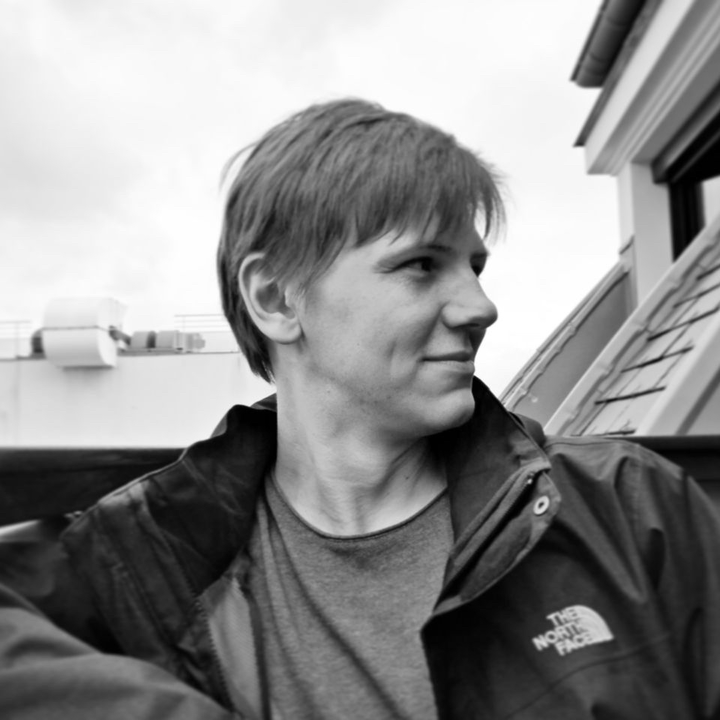# Talk of Nikita DoikovHello everyone,

On Monday, $23^{\text{rd}}$ September Nikita Doikov will give a talk about some recent results on accelerated proximal methods.

Nikita is a PhD student at Université Catholique de Louvain, Belgium, where he is working on developing high-order optimization methods in the group of Prof. Yurii Nesterov.

Title: Proximal Method with Contractions for Smooth Convex Optimization.

Abstract: In this work we study a construction of proximal accelerated methods for smooth convex optimization. At every step the method minimizes a sum of contracted objective and a regularizer in a form of Bregman divergence, by some auxiliary subroutine. We provide a simple practical stopping criterion for solving the subproblem and present complexity analysis for a general scheme. In the case when Tensor Method is used as internal method, we show accelerated rate of convergence in both convex and strongly convex composite cases with additional logarithmic factor as a cost for solving the subproblem.

Talk will take a place in Batiment IMAG in the room $\boldsymbol{106}$ (first floor) at $\boldsymbol{14^{00}}$ on Monday, $\boldsymbol{23^{\text{rd}}}$ September.

All are invited to attend.